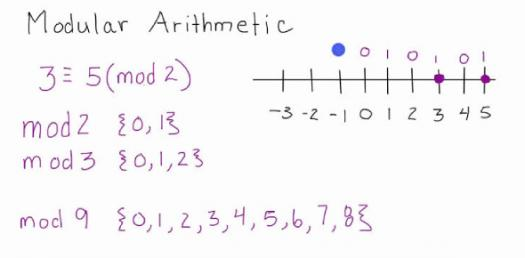# Ultimate Trivia Quiz On Arithmetic Progression: Can You Pass?

10 Questions | Attempts: 154
ShareSettingsCan you pass this ultimate trivia quiz on the arithmetic progression trivia quiz? There is a standardized formula for one to use when it comes to these types of math problems, and if you have a hard time solving them, then this quiz is perfect for you. Do give it a try and see how high you will score. All the best!

• 1.
Find the number of terms in series -10 , -7 ,-4 , ..... , 50.
• A.

20

• B.

22

• C.

21

• D.

15

• 2.
Find the 35th term of the AP  20 , 17, 14 , 11 , ....
• A.

-85

• B.

82

• C.

-80

• D.

-82

• 3.
How many terms are their in the AP 41 , 38 , 35 , ..... , 8?
• A.

18

• B.

12

• C.

15

• D.

10

• 4.
Which term of the AP 21 , 18 , 15 , .... is -81?
• A.

35th

• B.

36th

• C.

30th

• D.

32nd

• 5.
Find the 6th term from the end of the AP 17 , 14 , 11 , ...., (-40).
• A.

25

• B.

30

• C.

-35

• D.

-25

• 6.
The 4th term of an AP is 11. The sum of 5th and 7th terms of this AP is 34. Find its common difference.
• A.

5

• B.

3

• C.

2

• D.

-3

• 7.
Which term of the AP 121 , 117 , 113 , ... is its first negative term?
• A.

35th

• B.

36th

• C.

32nd

• D.

30th

• 8.
Is -150 a term of the AP 11 , 8 , 5 , 2 , ......?
• A.

Yes

• B.

NO

• 9.
How many two-digit numbers are divisible by 6?
• A.

14

• B.

20

• C.

16

• D.

15

• 10.
Find the value of p for which the numbers  2p -1 , 3p+1 , 11 are in AP ?
• A.

P=1

• B.

P=3

• C.

P=2

• D.

P= -2

## Related TopicsBack to top
×

Wait!
Here's an interesting quiz for you.